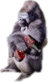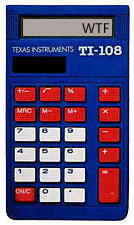# HowTo:Divide by Zero

(Redirected from How to Divide by Zero)HowToThis article is part of Uncyclopedia's HowTo series.See more HowTosChildren are born with the knowledge of division by zero, but it is forgotten after age 9This is the result of dividing by zero on a calculator.

Dividing by zero is a difficult feat. In fact, it's long been considered impossible, like licking your elbow. However, just as quadruple-amputees recently proved that licking one's own elbow is perfectly feasible, division by zero can also be accomplished.

One caveat: attempting this may cause the implosion of the universe.

## Okay.

Imagine a pie. No, no, that's a flan. Imagine a pie.

Good.

Now imagine that the pie is cut in two. That's how to divide by two.

Congratulations! Now you can divide by two! Now, imagine the pie is cut into slices a nothingth of an inch wide. They have no mass, area, or flavor. How many of these slices does it take to fill up the pie?

Forget about the pie. If you have imagined a nothingth of an inch, you have already divided something by zero! That wasn't so hard, now, was it?

Edit: A nothingth of an inch is actually zero divided by x, which would be zero. You actually have not imagined the division of zero, but only a division form of the zero-product property.

But how many slices, the value, are there when the pie is cut in zero?

Are you doing it?

Good.

Now, how many legs does a snake have?

That's right, none.

If an animal's weight distribution is it's mass divided by each leg- or limb, I suppose- then what is a snake's weight distribution?

A bear weighing 20kg has two legs 20kg÷2= 10kg.

A snake weighs 64kg. 64÷0= slither

So, the sound a snake makes is the consequence of dividing it's mass by 0 to achieve it's weight distribution.

Meaning a bear makes 10kg of noise.

Any questions?

Theoretically, there are range of answers when you divide something finite (Example: The PiCakeFlan, or whatever.) by zero. Possible values would be infinity, negative infinity, or some abstract value so powerful, perhaps even greater or equal to absolute infinity, that mathematicians couldn't define it in words. Mathematicians named this value Undefined or NaN, the latter especially when it is fresh out of the tandoori. While this value is "undefined" by definition, it is defined in IEEE floating-point arithmetic.

There were several attempts to define Undefined, but ultimately failed. One contributor, your mom, did help with long division homework once (Example: She divides you from your home to bring you to the mall, while you work boredom, or interest for long periods of time). But it wasn't enough to define Undefined, because it hides from your mom when going to the mall.

There's probability that some, or all of these answers are impossible to obtain in applied mathematics, as it may as well have been made up (Imaginary values). You want me to apply the real answer? Not until you've finished your pie. Hurry up and finish your pie!

Ok. Now, watch closely.

## Division by Zero and the End of the Universe

Some have theorized that a successful division by zero would lead to the creation of a new universe, which would annihilate the present universe. However, this may not be as problematic as it sounds, as the current universe is basically shite and the new one is likely to have free beer.

The Windows calculator bundled with Windows Vista is said to be able to divide a number by zero, but no one has been able to get past the billions and billions of warning prompts. Actually, they haven't even been able to get past the billions and billions of warning prompts required to open the calculator in the first place.

GNU EMACS can divide by zero, but the lisp function which does so recurs an infinite number of times because it is `strictfp`, requiring it to return an exact value, even when there is none.

The cost to the economy of not being able to divide by zero has been estimated to run into the billions of dollars, and has caused much snapping of pencils by apoplectic mathematicians/apprentice number-carriers.